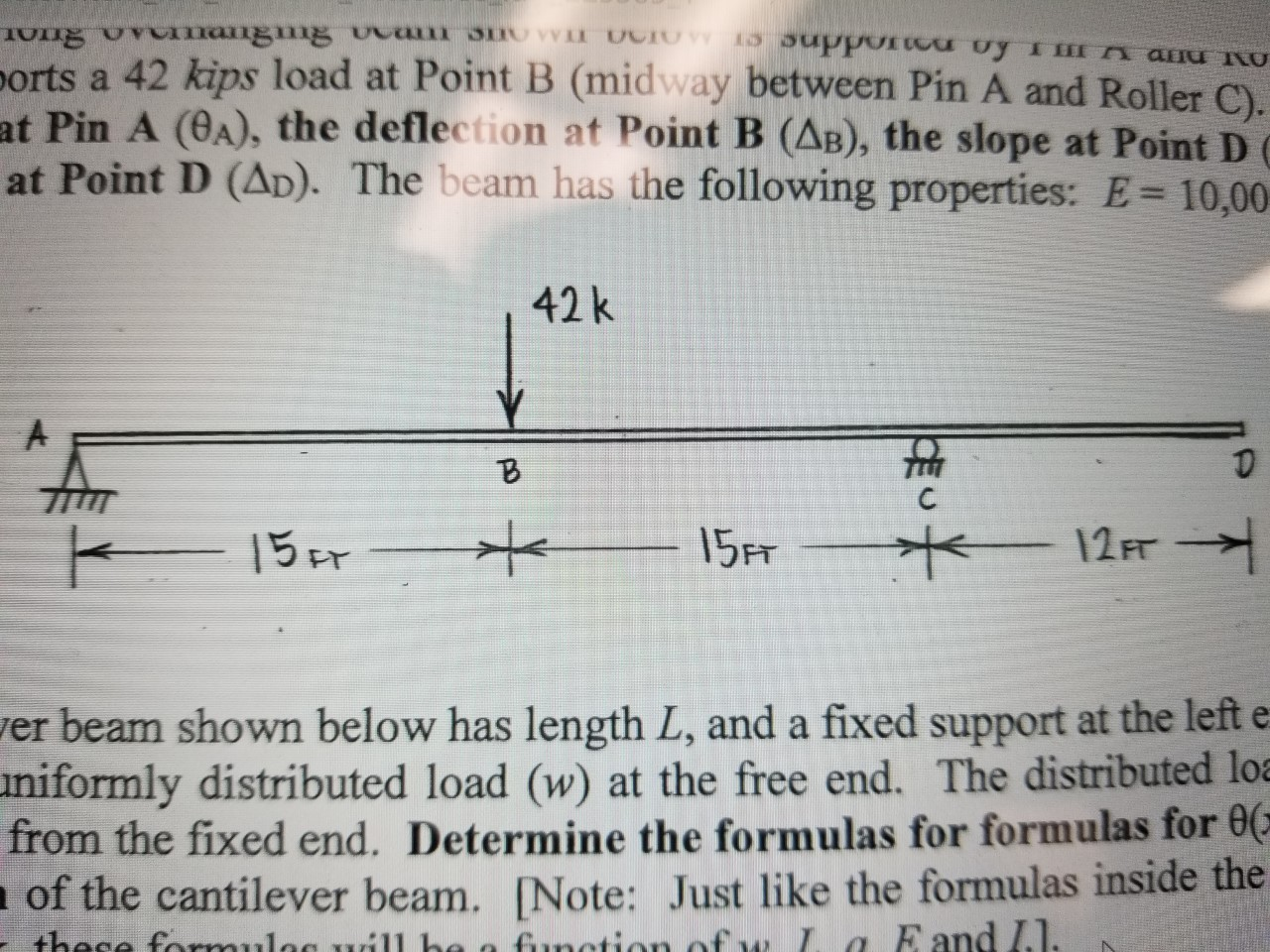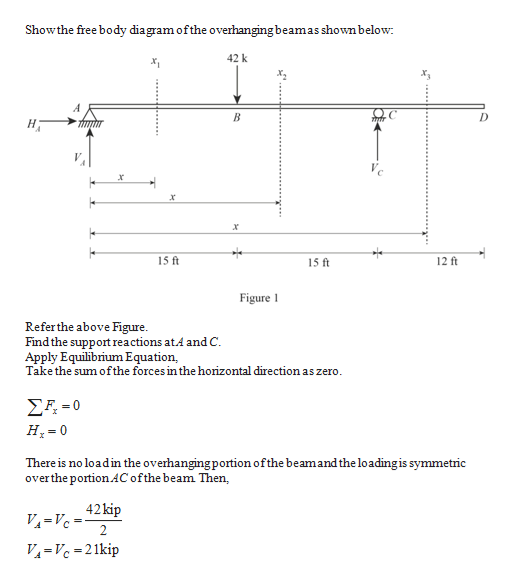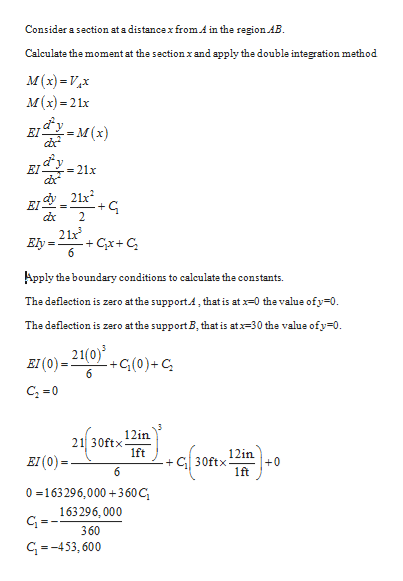# Om ang g caT VI CIO nPPOTcd Uy ImI an Uports a 42 kips load at Point B (midway between Pin A and Roller C).at Pin A (0A), the deflection at Point B (AB), the slope at Point D Cat Point D (AD). The beam has the following properties: E 10,0042k15FT15r12 FTer beam shown below has length L, and a fixed support at the left eniformly distributed load (w) at the free end. The distributed loafrom the fixed end. Determine the formulas for formulas for 0of the cantilever beam. [Note: Just like the formulas inside thefunction of w L a Eand I.1.+hese fo ues. he

Question

The 42 foot long overhanging beam shown is supported by a pin at A and a roller at C. The beam supports a point load of 42 kips at point B. Determine the slope at pin A, deflection at point B, and the slope and deflection at point D. Modulus of Elasticity, E = 10,000ksi, Moment of Inertia, I = 2500in4.

Let slope and deflection be functions of x, with x positive to the right.help_outlineImage TranscriptioncloseOm ang g caT VI CIO nPPOTcd Uy ImI an U ports a 42 kips load at Point B (midway between Pin A and Roller C). at Pin A (0A), the deflection at Point B (AB), the slope at Point D C at Point D (AD). The beam has the following properties: E 10,00 42k 15FT 15r 12 FT er beam shown below has length L, and a fixed support at the left e niformly distributed load (w) at the free end. The distributed loa from the fixed end. Determine the formulas for formulas for 0 of the cantilever beam. [Note: Just like the formulas inside the function of w L a Eand I.1. +hese fo ues. he fullscreen
check_circle

Step 1
Step 2help_outlineImage TranscriptioncloseShowthe free body diagram ofthe overhanging beamas shownbelow 42k ो н; 15 ft 12 ft 15 ft Figure Referthe above Figure. Find the support reactions atA and C. Apply Equilibrium Equation Take the sum ofthe forces in the horizontal direction as zero. ΣΕ-0 H 0 There is no loadin the overhangingportion ofthe beamand the loadingis symmetric overthe portionAC ofthe beam Then, 42kip V=Vc=2 V4-Vc=21kip fullscreen
Step 3help_outlineImage TranscriptioncloseConsider a section at a distance x from.A in the region AB. Calculate the moment at the sectionxand apply the double integration method. M(x)Vx м (х) -21х EIY M(x) EI - 21x cx 21x EI 2 21x +CxC 6 Еly Apply the boundary conditions to calculate the constants. The deflection is zero atthe supportA, thatis at -0 thevalue ofy-0. The deflection is zero atthe support B, that is atx-30 the value ofy 0. 21(0) +G(0)+ C 6 вI(0) - 12in 21 30ftx 1ft 12in EI(0)= +0 30ftx + 6 1ft 0 163296,000+3 60 C 163296, 000 360 G-453,600 n fullscreen

### Want to see the full answer?

See Solution

#### Want to see this answer and more?

Solutions are written by subject experts who are available 24/7. Questions are typically answered within 1 hour.*

See Solution
*Response times may vary by subject and question.
Tagged in

### Civil Engineering